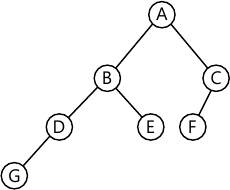MORE IN Data Structures and Applications
VTU Computer Science (Semester 3)
Data Structures and Applications
June 2012
Total marks: --
Total time: --
INSTRUCTIONS
(1) Assume appropriate data and state your reasons
(2) Marks are given to the right of every question
(3) Draw neat diagrams wherever necessary

1 (a) Define a pointer. Write a C function to swap two numbers using pointers.
5 M
1 (b) Explain the functions supported by C to carryout dynamic memory allocation.
5 M
1 (c) Explain performance analysis and performance measurement.
10 M

2 (a) Define structure and union with suitable example.
8 M
2 (b) Write a C program with an appropriate structure definition and variable declaration to store information about an employee using nested structures. Consider the following fields like Ename, Empid, DOJ (Date, Month, Year) and salary(Basic, DA, HRA).
12 M

3 (a) Write a C program to implement the two primite operations on stack using dynamic memory allocation.
8 M
3 (b) Write an algorithm to convert infix to postfix expression and apply the same to convert the following expression from infix to postfix:
i) (a*b)+c/d
ii) (((a/b)-c) + (d*e)) - (a*c)
12 M

4 (a) Define linked list. Write a C program to implement the insert and delete operation on a queue using linked list.
10 M
4 (b) Write a C function to add two polynomials using linked list representation. Explain with suitable example.
10 M

5 (a) Define binary trees. For the given tree find the following:

i) Siblings
ii) Leaf nodes
iii) Non-leaf nodes
iv) Ancestors
v) Level of trees.8 M
5 (b) Write the C routines to traverse the given tree using i) inorder; ii) pre order iii) posr order.
12 M

6 (a) Define ADT of binary search tree. Write the iterative search function and recursive search function of BST.
8 M
6 (b) Construct the binary tree for the given expression:
i) Pre order: / + * 1 $2 3 4 5 A B D G C E H I F In order: 1 + 2 * 3$ 4 - 5
D G B A H E I C F.
8 M
6 (c) Define furest with example.
4 M

7 (a) Define leftlist trees. Explain varieties of leftist trees.
8 M
7 (b) Write short notes on:
i) Priority queues
ii) Binomial heaps
iii) Priority heaps
iv) Fibonacci heaps.
12 M

8 (a) Define AVL trees. Write a C-routine for
i) Inserting into an AVL tree
ii) LL and LR rotation.
10 M
8 (b) Explain the following with example:
i) Red-black trees
ii) Splay trees.
10 M

More question papers from Data Structures and Applications